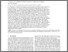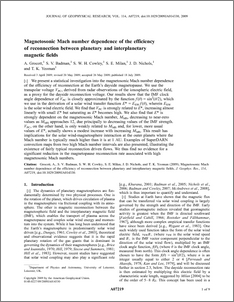# Magnetosonic Mach number dependence of the efficiency of reconnection between planetary and interplanetary magnetic fields

Grocott, Adrian and Badman, Sarah V. and Cowley, S.W.H. and Milan, S.E. and Nichols, J.D. and Yeoman, T.K. (2009) Magnetosonic Mach number dependence of the efficiency of reconnection between planetary and interplanetary magnetic fields. Journal of Geophysical Research, 114 (A7). ISSN 0148-0227Preview
PDF (jgra20006)
jgra20006.pdf - Published Version

We present a statistical investigation into the magnetosonic Mach number dependence of the efficiency of reconnection at the Earth's dayside magnetopause. We use the transpolar voltage V PC, derived from radar observations of the ionospheric electric field, as a proxy for the dayside reconnection voltage. Our results show that the IMF clock angle dependence of V PC is closely approximated by the function f($\theta$) = sin2($\theta$/2), which we use in the derivation of a solar wind transfer function E* = E SW f($\theta$), wherein E SW is the solar wind electric field. We find that V PC is strongly related to E*, increasing almost linearly with small E* but saturating as E* becomes high. We also find that E* is strongly dependent on the magnetosonic Mach number, M MS, decreasing to near-zero values as M MS approaches 12, due principally to decreasing values of the IMF strength. V PC, on the other hand, is only weakly related to M MS and, for lower, more usual values of E*, actually shows a modest increase with increasing M MS. This result has implications for the solar wind-magnetosphere interaction at the outer planets where the Mach number is typically much higher than it is at 1 AU. Examples of SuperDARN convection maps from two high Mach number intervals are also presented, illustrating the existence of fairly typical reconnection driven flows. We thus find no evidence for a significant reduction in the magnetopause reconnection rate associated with high magnetosonic Mach numbers.View Item